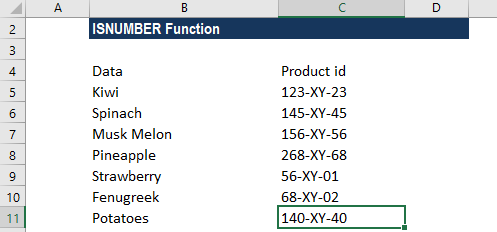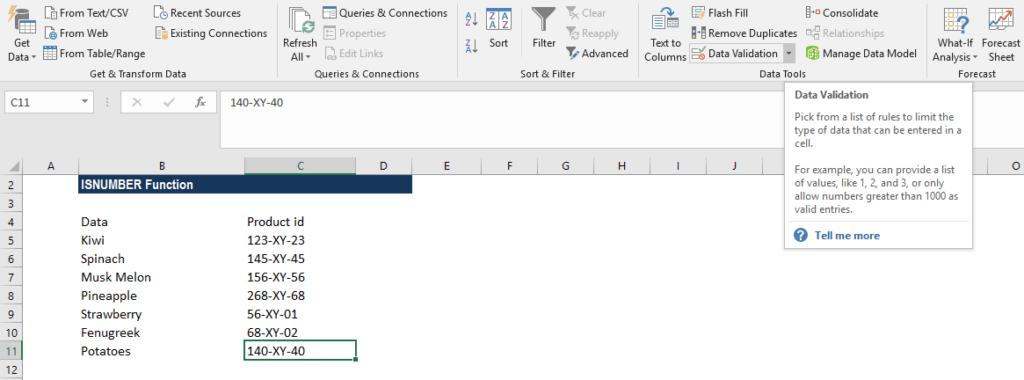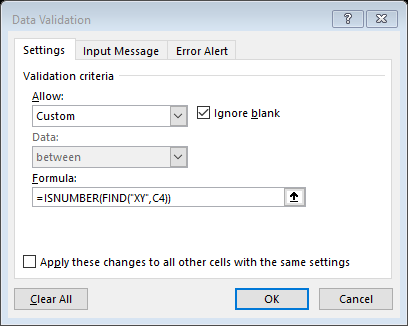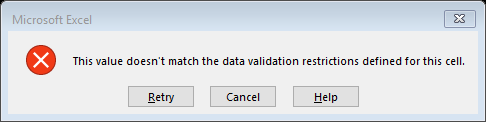# ISNUMBER Function

Checks if a cell contains a number or not

## What is the Excel ISNUMBER Function?

The Excel ISNUMBER function is categorized under Information functions. The function checks if a cell in Excel contains a number or not. It will return TRUE if the value is a number and if not, a FALSE value. For example, if the given value is a text, date, or time, it will return FALSE.

As a financial analyst, when dealing with large amounts of data, ISNUMBER Excel function helps in testing if a given result of a formula is a number or not.

### Formula

=ISNUMBER(value)

The Excel ISNUMBER function uses the following arguments:

1. Value (required argument) – This is the expression or value that needs to be tested. It is generally provided as a cell address.

The ISNUMBER Excel function will return a logical value, which is TRUE or FALSE.

### How to use the ISNUMBER Excel Function?

To understand the uses of the ISNUMBER Excel function, let’s consider a few examples:

#### Example 1

Let’s first understand how the function behaves using the following set of data:

DataFormulaResultRemark
1=ISNUMBER(1)TRUEThe value provided is a number, so the function returned TRUE.
TEXT=ISNUMBER(TEXT)FALSEThe function returns FALSE for text values.
10/20=ISNUMBER(10/20)TRUEThe formula will return a number, so the function returned TRUE.
#NAME?=ISNUMBER(#NAME?)FALSEThe function returned FALSE for formula errors.
=ISNUMBER( )FALSEThe result is FALSE, as it is not a number.

#### Example 2 – Check if Excel contains a number in a cell

Suppose we wish to allow values that contain the text string XY. Using ISNUMBER along with data validation, we can check if Excel contains “XY” in the cell. Suppose we are given the following data:To allow only values that contain a specific text string, we can use data validation with a customized formula based on the FIND and ISNUMBER Excel functions. Essentially, it checks if Excel contains a number in the cell or not. To do this, we will apply data validation to C4:C11 cells. To apply the data validation, click on the Data tab, go to Data Tools, and click on Data Validation, as shown below:Now, select Data validation and click on Settings. As shown below, enter the formula =ISNUMBER(FIND(“XY”,C4)). To activate the formula cell, you need to choose Validation criteria – Allow – Custom.When someone tries to change XY into something else, data validation rules are triggered, particularly when a user adds or changes a cell value.The FIND function is shaped to search for the text “XY” in cell C4. If found, FIND will return a numeric position (e.g., 2, 4, 5, etc.) for the starting point of the text in the cell. If the text is not found, FIND will return an error. For C4, FIND will return 5, since “XY” starts at character 5.

The result from using the FIND function above is then evaluated by the ISNUMBER Excel function. For any numeric result returned by FIND, ISNUMBER will return TRUE and validation will succeed. When text is not found, FIND will return an error, ISNUMBER will return FALSE, and the input will fail validation.

### A few notes about the ISNUMBER Function

1. The function is part of the IS functions that return the logical values TRUE or FALSE.
2. The function doesn’t return any error such as #NAME!, #N/A!, etc., as it just evaluates data.
3. The function was introduced in MS Excel 2000.

Thanks for reading CFI’s guide to important Excel functions! By taking the time to learn and master these functions, you’ll significantly speed up your financial analysis. To learn more, check out these additional CFI resources:

• Excel Functions for Finance